# ASH-based wavelet shrinkage for heteroskedastic models

#### 2018-05-21

In this vignette, we assume a simple parametric model of the form

$y_i = \mu_i + \epsilon_i,$

where $$\epsilon_i \sim N(0,\sigma_i^2)$$, and both $$\mu$$ and $$\sigma$$ are unknowns. We use the “smash” procedure (SMoothing via Adaptive SHrinkage) to estimate both the mean and the variances. Here we present a brief demonstration of the method.

We first look at mean estimation, which is our primary focus. A sample mean function is presented, as well as a couple of different variance functions. Our method is compared against a few other simple methods.

## Set up environment

library(MASS)
library(smashr)
library(EbayesThresh)
library(wavethresh)

## Preparations

First, we define the mean function used to simulate the data.

spike.f <- function(x) (0.75 * exp(-500 * (x - 0.23)^2) +
1.5  * exp(-2000  * (x - 0.33)^2) +
3    * exp(-8000  * (x - 0.47)^2) +
2.25 * exp(-16000 * (x - 0.69)^2) +
0.5  * exp(-32000 * (x - 0.83)^2))
n    <- 1024
t    <- 1:n/n
mu.s <- spike.f(t)

Define a few other functions which will be used in the code chunks below.

mse    <- function(x, y) mean((x - y)^2)
l2norm <- function(x) sum(x^2)
mise   <- function(x, y, r)
10000 * mean(apply(x - rep(1, r) %o% y, 1, l2norm)/l2norm(y))
sig.est.func <- function(x, n)
sqrt(2/(3 * (n - 2)) * sum((1/2 * x[1:(n - 2)] - x[2:(n - 1)] +
1/2 * x[3:n])^2))

Define a function for the default wavelet thresholding method.

waveti.u <- function(x, filter.number = 10, family = "DaubLeAsymm",
min.level = 3, noise.level) {
TT = length(x)
thresh = noise.level * sqrt(2 * log(TT))
x.w = wavethresh::wd(x, filter.number, family, type = "station")
x.w.t = threshold(x.w, levels = (min.level):(x.w$nlevels - 1), policy = "manual", value = thresh, type = "hard") x.w.t.r = AvBasis(convert(x.w.t)) return(x.w.t.r) } Define another function for the default EbayesThresh method. waveti.ebayes <- function(x, filter.number = 10, family = "DaubLeAsymm", min.level = 3, noise.level) { n = length(x) J = log2(n) x.w = wd(x, filter.number, family, type = "station") for (j in min.level:(J - 1)) { x.pm = ebayesthresh(accessD(x.w, j), sdev = noise.level) x.w = putD(x.w, j, x.pm) } mu.est = AvBasis(convert(x.w)) return(mu.est) } For the first demonstration, define the mean and variance functions, and set the signal to noise ratio. mu.t <- (1 + mu.s)/5 rsnr <- sqrt(1) var1 <- rep(1, n) var2 <- (1e-04 + 4 * (exp(-550 * (t - 0.2)^2) + exp(-200 * (t - 0.5)^2) + exp(-950 * (t - 0.8)^2)))/1.35  ## Constant variance example We first look at the case of constant variance. set.seed(327) sigma.ini <- sqrt(var1) sigma.t <- sigma.ini/mean(sigma.ini) * sd(mu.t)/rsnr^2 X.s <- matrix(rnorm(10 * n, mu.t, sigma.t), nrow = 10, byrow = TRUE) mu.est <- apply(X.s, 1, smash.gaus) mu.est.tivar.ash <- apply(X.s, 1, ti.thresh, method = "smash") mu.est.tivar.mad <- apply(X.s, 1, ti.thresh, method = "rmad") mu.est.ti <- matrix(0, 10, n) mu.est.ti.ebayes <- matrix(0, 10, n) for (i in 1:10) { sig.est = sig.est.func(X.s[i, ], n) mu.est.ti[i, ] = waveti.u(X.s[i, ], noise.level = sig.est) mu.est.ti.ebayes[i, ] = waveti.ebayes(X.s[i, ], noise.level = sig.est) } Assess the accuracy of the results. cat("SMASH:",mise(t(mu.est), mu.t, 10),"\n") cat("TI thresholding with variance estimated from smash:", mise(t(mu.est.tivar.ash), mu.t, 10),"\n") cat("TI thresholding with variance estimated from running MAD:", mise(t(mu.est.tivar.mad), mu.t, 10),"\n") cat("TI thresholding with constant variance (estimated):", mise(mu.est.ti, mu.t, 10),"\n") cat("EBayes with constant variance (estimated):", mise(mu.est.ti.ebayes, mu.t, 10),"\n") # SMASH: 59.77 # TI thresholding with variance estimated from smash: 78.47 # TI thresholding with variance estimated from running MAD: 118.9 # TI thresholding with constant variance (estimated): 87.7 # EBayes with constant variance (estimated): 75.02 Plot the estimated mean functions against the ground-truth function (in black). plot(mu.t, xlab = "", ylab = "", type = "l") lines(mu.est[, 1], col = 2) lines(mu.est.tivar.mad[, 1], col = 3) lines(mu.est.ti[1, ], col = 4) lines(mu.est.ti.ebayes[1, ], col = 6) legend("topright", legend = c("smash", "ti_rmad", "ti_homo", "ebayes_homo"), fill = c(2, 3, 4, 6))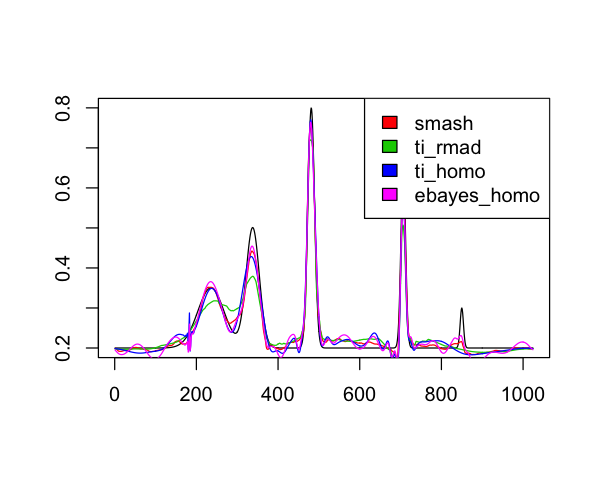## Non-constant variance example Generate the data for this example. sigma.ini = sqrt(var2) sigma.t = sigma.ini/mean(sigma.ini) * sd(mu.t)/rsnr^2 set.seed(327) X.s = matrix(rnorm(10 * n, mu.t, sigma.t), nrow = 10, byrow = TRUE) mu.est = apply(X.s, 1, smash.gaus) mu.est.tivar.ash = apply(X.s, 1, ti.thresh, method = "smash") mu.est.tivar.mad = apply(X.s, 1, ti.thresh, method = "rmad") mu.est.ti = matrix(0, 10, n) mu.est.ti.ebayes = matrix(0, 10, n) for (i in 1:10) { sig.est = sig.est.func(X.s[i, ], n) mu.est.ti[i, ] = waveti.u(X.s[i, ], noise.level = sig.est) mu.est.ti.ebayes[i, ] = waveti.ebayes(X.s[i, ], noise.level = sig.est) } Assess the accuracy of the results. cat("SMASH:",mise(t(mu.est), mu.t, 10),"\n") cat("TI thresholding with variance estimated from smash:", mise(t(mu.est.tivar.ash), mu.t, 10),"\n") cat("TI thresholding with variance estimated from running MAD:", mise(t(mu.est.tivar.mad), mu.t, 10),"\n") cat("TI thresholding with constant variance (estimated):", mise(mu.est.ti, mu.t, 10),"\n") cat("EBayes with constant variance (estimated):", mise(mu.est.ti.ebayes, mu.t, 10) ,"\n") # SMASH: 106.9 # TI thresholding with variance estimated from smash: 141.9 # TI thresholding with variance estimated from running MAD: 215.7 # TI thresholding with constant variance (estimated): 400.4 # EBayes with constant variance (estimated): 431.2 Plot the estimated mean functions against the ground-truth function (in black). plot(mu.t, xlab = "", ylab = "", type = "l") lines(mu.est[, 1], col = 2) lines(mu.est.tivar.mad[, 1], col = 3) lines(mu.est.ti[1, ], col = 4) lines(mu.est.ti.ebayes[1, ], col = 6) legend("topright", legend = c("smash", "ti_rmad", "ti_homo", "ebayes_homo"), fill = c(2, 3, 4, 6))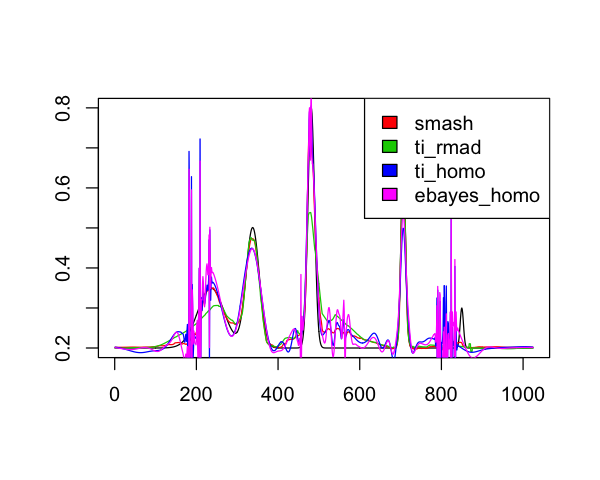## Estimating the variance function Next we look at variance estimation. Because variance estimation is much harder, we use a larger sample size. In this example, we set $$n = 4096$$. n = 2^12 t = 1:n/n mu.s = spike.f(t) Define the test functions and generate the data. pos = c(0.1, 0.13, 0.15, 0.23, 0.25, 0.4, 0.44, 0.65, 0.76, 0.78, 0.81) hgt = c(4, 5, 3, 4, 5, 4.2, 2.1, 4.3, 3.1, 5.1, 4.2) wth = c(0.005, 0.005, 0.006, 0.01, 0.01, 0.03, 0.01, 0.01, 0.005, 0.008, 0.005) mu.b = rep(0, n) for (j in 1:length(pos)) { mu.b = mu.b + hgt[j]/((1 + (abs(t - pos[j])/wth[j]))^4) } dop.f = function(x) sqrt(x * (1 - x)) * sin((2 * pi * 1.05)/(x + 0.05)) mu.dop = dop.f(t) var1.ini = ((3 - 20 * t) * (t >= 0 & t < 0.1) + (20 * t - 1) * (t >= 0.1 & t < 0.25) + (4 + (1 - 4 * t) * 18/19) * (t >= 0.25 & t < 0.725) + (2.2 + 10 * (t - 0.725)) * (t >= 0.725 & t < 0.89) + (3.85 - 85 * (t - 0.89)/11) * (t >= 0.89 & t <= 1)) var1 = var1.ini/sqrt(var(var1.ini)) var2.ini = (1 + 4 * (exp(-550 * (t - 0.2)^2) + exp(-200 * (t - 0.5)^2) + exp(-950 * (t - 0.8)^2))) var2 = var2.ini/sqrt(var(var2.ini)) var3 = mu.b/sqrt(var(mu.b)) var4 = (mu.dop + 2)/sqrt(var(mu.dop)) sigma.t.1 = sqrt(var1) sigma.t.2 = sqrt(var2) sigma.t.3 = sqrt(var3) sigma.t.4 = sqrt(var4) set.seed(327) X.s.1 = rnorm(n, 0, sigma.t.1) set.seed(327) X.s.2 = rnorm(n, 0, sigma.t.2) set.seed(327) X.s.3 = rnorm(n, 0, sigma.t.3) set.seed(327) X.s.4 = rnorm(n, 0, sigma.t.4) Fit the SMASH model to these data. var.est.1 = smash.gaus(X.s.1, v.est = TRUE) var.est.2 = smash.gaus(X.s.2, v.est = TRUE) var.est.3 = smash.gaus(X.s.3, v.est = TRUE) var.est.4 = smash.gaus(X.s.4, v.est = TRUE) Evaluate the fit of these models. mse(var.est.1, sigma.t.1^2) mse(var.est.2, sigma.t.2^2) mse(var.est.3, sigma.t.3^2) mse(var.est.4, sigma.t.4^2) #  0.09105 #  0.0551 #  0.297 #  0.5154 These plots show the ground-truth variance (black) against the variance functions estimated by SMASH (red). par(mfrow = c(2, 2)) plot(sigma.t.1^2, xlab = "", ylab = "", type = "l", main = "V1") lines(var.est.1, col = 2) plot(sigma.t.2^2, xlab = "", ylab = "", type = "l", main = "V2") lines(var.est.2, col = 2) plot(sigma.t.3^2, xlab = "", ylab = "", type = "l", main = "V3") lines(var.est.3, col = 2) plot(sigma.t.4^2, xlab = "", ylab = "", type = "l", main = "V4") lines(var.est.4, col = 2)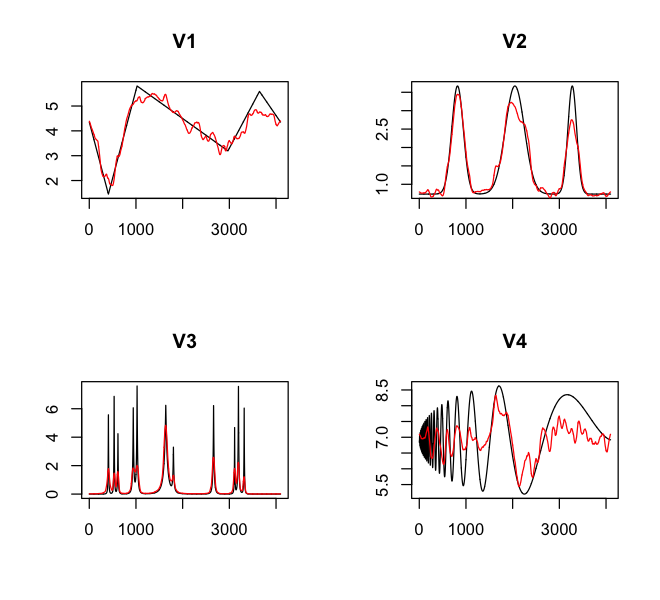## Unevenly spaced data In this section, we examine how this wavelet-based approach handles unevenly spaced data. Wavelet methods are not optimized for unevenly spaced data, but still works. We first look at the case when the data are normally spaced. n = 1024 t = sort(rnorm(1024, 0, 1)) t = (t - min(t))/(max(t) - min(t)) mu.s = spike.f(t) Define mean function, signal-to-noise ratio, and the variance functions. mu.t = (1 + mu.s)/5 rsnr = sqrt(1) var1 = rep(1, n) var2 = (1e-04 + 4 * (exp(-550 * (t - 0.2)^2) + exp(-200 * (t - 0.5)^2) + exp(-950 * (t - 0.8)^2)))/1.35 ### Unevenly spaced data with constant variance First, we look at the case of constant variance. sigma.ini = sqrt(var1) sigma.t = sigma.ini/mean(sigma.ini) * sd(mu.t)/rsnr^2 set.seed(327) X.s = matrix(rnorm(10 * n, mu.t, sigma.t), nrow = 10, byrow = TRUE) Apply the methods on the data. mu.est = apply(X.s, 1, smash.gaus) mu.est.tivar.ash = apply(X.s, 1, ti.thresh, method = "smash") mu.est.tivar.mad = apply(X.s, 1, ti.thresh, method = "rmad") mu.est.ti = matrix(0, 10, n) mu.est.ti.ebayes = matrix(0, 10, n) for (i in 1:10) { sig.est = sig.est.func(X.s[i, ], n) mu.est.ti[i, ] = waveti.u(X.s[i, ], noise.level = sig.est) mu.est.ti.ebayes[i, ] = waveti.ebayes(X.s[i, ], noise.level = sig.est) } Assess the accuracy of the estimates. cat("SMASH:",mise(t(mu.est), mu.t, 10),"\n") cat("TI thresholding with variance estimated from smash:", mise(t(mu.est.tivar.ash), mu.t, 10),"\n") cat("TI thresholding with variance estimated from running MAD:", mise(t(mu.est.tivar.mad), mu.t, 10),"\n") cat("TI thresholding with constant variance (estimated):", mise(mu.est.ti, mu.t, 10),"\n") cat("EBayes with constant variance (estimated):", mise(mu.est.ti.ebayes, mu.t, 10),"\n") # SMASH: 82.93 # TI thresholding with variance estimated from smash: 122.7 # TI thresholding with variance estimated from running MAD: 182.7 # TI thresholding with constant variance (estimated): 110.9 # EBayes with constant variance (estimated): 87.52 Assess the accuracy over a grid. xgrid = 1:n/n mu.est.inter = matrix(0, 10, n) mu.est.tivar.ash.inter = matrix(0, 10, n) mu.est.tivar.mad.inter = matrix(0, 10, n) mu.est.ti.inter = matrix(0, 10, n) mu.est.ti.ebayes.inter = matrix(0, 10, n) for (i in 1:10) { mu.est.inter[i, ] = approx(t, mu.est[, i], xgrid)$y
mu.est.tivar.ash.inter[i, ] = approx(t, mu.est.tivar.ash[, i], xgrid)$y mu.est.tivar.mad.inter[i, ] = approx(t, mu.est.tivar.mad[, i], xgrid)$y
mu.est.ti.inter[i, ] = approx(t, mu.est.ti[i, ], xgrid)$y mu.est.ti.ebayes.inter[i, ] = approx(t, mu.est.ti.ebayes[i, ], xgrid)$y

}
mu.t.inter = (spike.f(xgrid) + 1)/5
mise(mu.est.inter, mu.t.inter, 10)
mise(mu.est.tivar.ash.inter, mu.t.inter, 10)
mise(mu.est.ti.inter, mu.t.inter, 10)
mise(mu.est.ti.ebayes.inter, mu.t.inter, 10)
#  167.3
#  298.8
#  424.1
#  229.3
#  141.8

Plot the estimated mean functions against the ground-truth function (in black).

par(mfrow = c(1, 1))
plot(t, mu.t, type = "l")
lines(t, mu.est[, 1], col = 2)
lines(t, mu.est.tivar.mad[, 1], col = 3)
lines(t, mu.est.ti[1, ], col = 4)
lines(t, mu.est.ti.ebayes[1, ], col = 6)
legend("topright", legend = c("smash", "ti_rmad", "ti_homo", "ebayes_homo"),
fill = c(2, 3, 4, 6))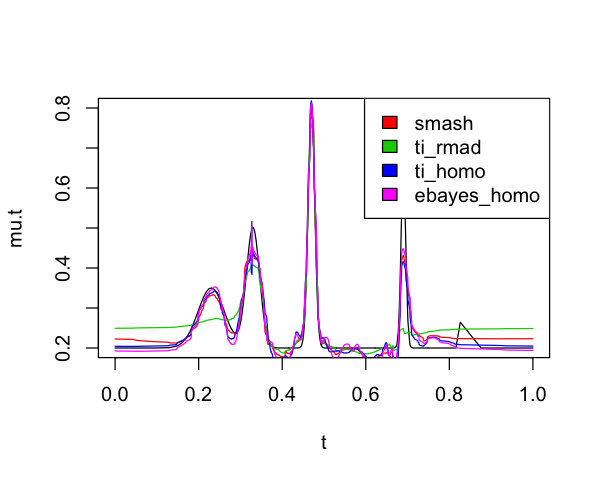### Unevenly spaced data with non-constant variance

We then look at the case of non-constant variance:

sigma.ini = sqrt(var2)
sigma.t = sigma.ini/mean(sigma.ini) * sd(mu.t)/rsnr^2
set.seed(327)
X.s = matrix(rnorm(10 * n, mu.t, sigma.t), nrow = 10, byrow = TRUE)
mu.est = apply(X.s, 1, smash.gaus)
mu.est.tivar.ash = apply(X.s, 1, ti.thresh, method = "smash")
mu.est.ti = matrix(0, 10, n)
mu.est.ti.ebayes = matrix(0, 10, n)
for (i in 1:10) {
sig.est = sig.est.func(X.s[i, ], n)
mu.est.ti[i, ] = waveti.u(X.s[i, ], noise.level = sig.est)
mu.est.ti.ebayes[i, ] = waveti.ebayes(X.s[i, ], noise.level = sig.est)
}

Assess the accuracy of the estimates.

cat("SMASH:",mise(t(mu.est), mu.t, 10),"\n")
cat("TI thresholding with variance estimated from smash:",
mise(t(mu.est.tivar.ash), mu.t, 10),"\n")
cat("TI thresholding with variance estimated from running MAD:",
cat("TI thresholding with constant variance (estimated):",
mise(mu.est.ti, mu.t, 10),"\n")
cat("EBayes with constant variance (estimated):",
mise(mu.est.ti.ebayes, mu.t, 10),"\n")
# SMASH: 95.97
# TI thresholding with variance estimated from smash: 126.4
# TI thresholding with variance estimated from running MAD: 209.7
# TI thresholding with constant variance (estimated): 305.8
# EBayes with constant variance (estimated): 295.4

Assess the accuracy over a grid.

xgrid = 1:n/n
mu.est.inter = matrix(0, 10, n)
mu.est.tivar.ash.inter = matrix(0, 10, n)
mu.est.ti.inter = matrix(0, 10, n)
mu.est.ti.ebayes.inter = matrix(0, 10, n)
for (i in 1:10) {
mu.est.inter[i, ] = approx(t, mu.est[, i], xgrid)$y mu.est.tivar.ash.inter[i, ] = approx(t, mu.est.tivar.ash[, i], xgrid)$y
mu.est.tivar.mad.inter[i, ] = approx(t, mu.est.tivar.mad[, i], xgrid)$y mu.est.ti.inter[i, ] = approx(t, mu.est.ti[i, ], xgrid)$y
mu.est.ti.ebayes.inter[i, ] = approx(t, mu.est.ti.ebayes[i, ], xgrid)$y } mu.t.inter = (spike.f(xgrid) + 1)/5 mise(mu.est.inter, mu.t.inter, 10) mise(mu.est.tivar.ash.inter, mu.t.inter, 10) mise(mu.est.tivar.mad.inter, mu.t.inter, 10) mise(mu.est.ti.inter, mu.t.inter, 10) mise(mu.est.ti.ebayes.inter, mu.t.inter, 10) #  155.1 #  252 #  443.6 #  398.2 #  302.2 Plot the estimated mean functions against the ground-truth function (in black). par(mfrow = c(1, 1)) plot(t, mu.t, xlab = "", ylab = "", type = "l") lines(t, mu.est[, 1], col = 2) lines(t, mu.est.tivar.mad[, 1], col = 3) lines(t, mu.est.ti[1, ], col = 4) lines(t, mu.est.ti.ebayes[1, ], col = 6) legend("topright", legend = c("smash", "ti_rmad", "ti_homo", "ebayes_homo"), fill = c(2, 3, 4, 6))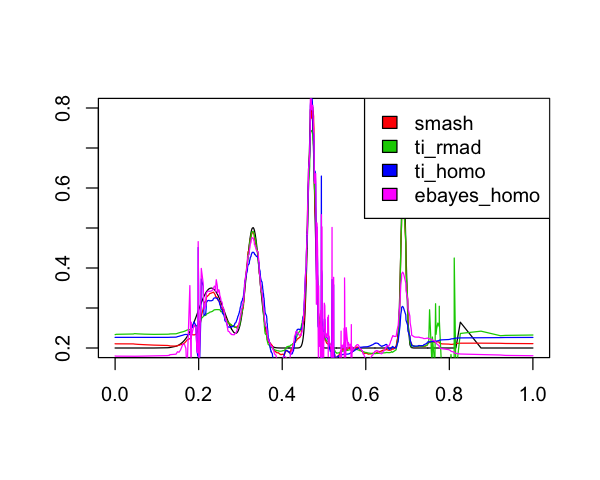## Poisson-distributed data example Now we consider the case in which the data are distributed according to a Poisson process. n <- 1024 t <- c(0, rexp(n - 1, 1)) t <- cumsum(t) t <- (t - min(t))/(max(t) - min(t)) mu.s <- spike.f(t) Next, define the mean and variance functions. mu.t <- (1 + mu.s)/5 rsnr <- sqrt(1) var1 <- rep(1, n) var2 <- (1e-04 + 4 * (exp(-550 * (t - 0.2)^2) + exp(-200 * (t - 0.5)^2) + exp(-950 * (t - 0.8)^2)))/1.35  ### Constant variance We first look at the case of constant variance. sigma.ini = sqrt(var1) sigma.t = sigma.ini/mean(sigma.ini) * sd(mu.t)/rsnr^2 set.seed(327) X.s = matrix(rnorm(10 * n, mu.t, sigma.t), nrow = 10, byrow = TRUE) mu.est = apply(X.s, 1, smash.gaus) mu.est.tivar.ash = apply(X.s, 1, ti.thresh, method = "smash") mu.est.tivar.mad = apply(X.s, 1, ti.thresh, method = "rmad") mu.est.ti = matrix(0, 10, n) mu.est.ti.ebayes = matrix(0, 10, n) for (i in 1:10) { sig.est = sig.est.func(X.s[i, ], n) mu.est.ti[i, ] = waveti.u(X.s[i, ], noise.level = sig.est) mu.est.ti.ebayes[i, ] = waveti.ebayes(X.s[i, ], noise.level = sig.est) } Assess accuracy of the results. cat("SMASH:",mise(t(mu.est), mu.t, 10),"\n") cat("TI thresholding with variance estimated from smash:", mise(t(mu.est.tivar.ash), mu.t, 10),"\n") cat("TI thresholding with variance estimated from running MAD:", mise(t(mu.est.tivar.mad), mu.t, 10),"\n") cat("TI thresholding with constant variance (estimated):", mise(mu.est.ti, mu.t, 10),"\n") cat("EBayes with constant variance (estimated):", mise(mu.est.ti.ebayes, mu.t, 10),"\n") # SMASH: 59.59 # TI thresholding with variance estimated from smash: 75.22 # TI thresholding with variance estimated from running MAD: 106 # TI thresholding with constant variance (estimated): 83.44 # EBayes with constant variance (estimated): 73.97 Assess accuracy over a grid. xgrid = 1:n/n mu.est.inter = matrix(0, 10, n) mu.est.tivar.ash.inter = matrix(0, 10, n) mu.est.tivar.mad.inter = matrix(0, 10, n) mu.est.ti.inter = matrix(0, 10, n) mu.est.ti.ebayes.inter = matrix(0, 10, n) for (i in 1:10) { mu.est.inter[i, ] = approx(t, mu.est[, i], xgrid)$y
mu.est.tivar.ash.inter[i, ] = approx(t, mu.est.tivar.ash[, i], xgrid)$y mu.est.tivar.mad.inter[i, ] = approx(t, mu.est.tivar.mad[, i], xgrid)$y
mu.est.ti.inter[i, ] = approx(t, mu.est.ti[i, ], xgrid)$y mu.est.ti.ebayes.inter[i, ] = approx(t, mu.est.ti.ebayes[i, ], xgrid)$y
}
mu.t.inter = (spike.f(xgrid) + 1)/5
mise(mu.est.inter, mu.t.inter, 10)
mise(mu.est.tivar.ash.inter, mu.t.inter, 10)
mise(mu.est.ti.inter, mu.t.inter, 10)
mise(mu.est.ti.ebayes.inter, mu.t.inter, 10)
#  65.54
#  83.59
#  120.9
#  87.98
#  79.18

Plot the estimated mean functions against the ground-truth function (in black).

par(mfrow = c(1, 1))
plot(t, mu.t, xlab = "", ylab = "", type = "l")
lines(t, mu.est[, 1], col = 2)
lines(t, mu.est.tivar.mad[, 1], col = 3)
lines(t, mu.est.ti[1, ], col = 4)
lines(t, mu.est.ti.ebayes[1, ], col = 6)
legend("topright",legend = c("smash", "ti_rmad", "ti_homo", "ebayes_homo"),
fill = c(2, 3, 4, 6))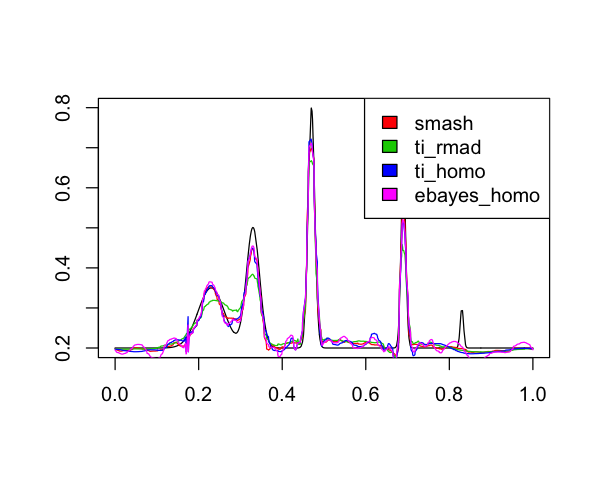### Non-constant variance

We then look at the case of non-constant variance.

sigma.ini = sqrt(var2)
sigma.t = sigma.ini/mean(sigma.ini) * sd(mu.t)/rsnr^2
set.seed(327)
X.s = matrix(rnorm(10 * n, mu.t, sigma.t), nrow = 10, byrow = TRUE)
mu.est = apply(X.s, 1, smash.gaus)
mu.est.tivar.ash = apply(X.s, 1, ti.thresh, method = "smash")
mu.est.ti = matrix(0, 10, n)
mu.est.ti.ebayes = matrix(0, 10, n)
for (i in 1:10) {
sig.est = sig.est.func(X.s[i, ], n)
mu.est.ti[i, ] = waveti.u(X.s[i, ], noise.level = sig.est)
mu.est.ti.ebayes[i, ] = waveti.ebayes(X.s[i, ], noise.level = sig.est)
}

Assess accuracy of the results.

cat("SMASH:",mise(t(mu.est), mu.t, 10),"\n")
cat("TI thresholding with variance estimated from smash:",
mise(t(mu.est.tivar.ash), mu.t, 10),"\n")
cat("TI thresholding with variance estimated from running MAD:",
cat("TI thresholding with constant variance (estimated):",
mise(mu.est.ti, mu.t, 10),"\n")
cat("EBayes with constant variance (estimated):",
mise(mu.est.ti.ebayes, mu.t, 10),"\n")
# SMASH: 105.8
# TI thresholding with variance estimated from smash: 138.5
# TI thresholding with variance estimated from running MAD: 213.2
# TI thresholding with constant variance (estimated): 362.6
# EBayes with constant variance (estimated): 385.4

Assess accuracy over a grid.

xgrid = 1:n/n
mu.est.inter = matrix(0, 10, n)
mu.est.tivar.ash.inter = matrix(0, 10, n)
mu.est.ti.inter = matrix(0, 10, n)
mu.est.ti.ebayes.inter = matrix(0, 10, n)
for (i in 1:10) {
mu.est.inter[i, ] = approx(t, mu.est[, i], xgrid)$y mu.est.tivar.ash.inter[i, ] = approx(t, mu.est.tivar.ash[, i], xgrid)$y
mu.est.tivar.mad.inter[i, ] = approx(t, mu.est.tivar.mad[, i], xgrid)$y mu.est.ti.inter[i, ] = approx(t, mu.est.ti[i, ], xgrid)$y
var.est.inter = approx(x, var.est, xgrid, "linear")$y mu.mad[i] = 1/101 * sum(abs(mu.est.inter - mu.t.inter)) var.mad[i] = 1/101 * sum(abs(var.est.inter - var.t.inter)) } cat("\n") # Running 400 simulations. # 1 2 3 4 5 6 7 8 9 10 11 12 13 14 15 16 17 18 19 20 21 22 23 24 25 26 27 28 29 30 31 32 33 34 35 36 37 38 39 40 41 42 43 44 45 46 47 48 49 50 51 52 53 54 55 56 57 58 59 60 61 62 63 64 65 66 67 68 69 70 71 72 73 74 75 76 77 78 79 80 81 82 83 84 85 86 87 88 89 90 91 92 93 94 95 96 97 98 99 100 101 102 103 104 105 106 107 108 109 110 111 112 113 114 115 116 117 118 119 120 121 122 123 124 125 126 127 128 129 130 131 132 133 134 135 136 137 138 139 140 141 142 143 144 145 146 147 148 149 150 151 152 153 154 155 156 157 158 159 160 161 162 163 164 165 166 167 168 169 170 171 172 173 174 175 176 177 178 179 180 181 182 183 184 185 186 187 188 189 190 191 192 193 194 195 196 197 198 199 200 201 202 203 204 205 206 207 208 209 210 211 212 213 214 215 216 217 218 219 220 221 222 223 224 225 226 227 228 229 230 231 232 233 234 235 236 237 238 239 240 241 242 243 244 245 246 247 248 249 250 251 252 253 254 255 256 257 258 259 260 261 262 263 264 265 266 267 268 269 270 271 272 273 274 275 276 277 278 279 280 281 282 283 284 285 286 287 288 289 290 291 292 293 294 295 296 297 298 299 300 301 302 303 304 305 306 307 308 309 310 311 312 313 314 315 316 317 318 319 320 321 322 323 324 325 326 327 328 329 330 331 332 333 334 335 336 337 338 339 340 341 342 343 344 345 346 347 348 349 350 351 352 353 354 355 356 357 358 359 360 361 362 363 364 365 366 367 368 369 370 371 372 373 374 375 376 377 378 379 380 381 382 383 384 385 386 387 388 389 390 391 392 393 394 395 396 397 398 399 400 Show the model fitting results. boxplot(var.mad)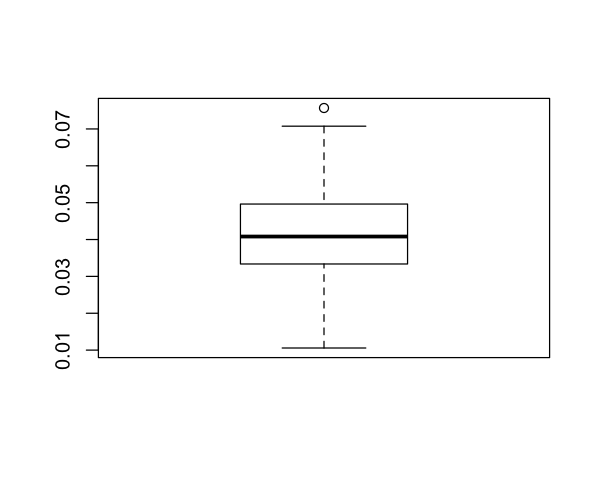plot(x, mu.t, xlab = "", ylab = "", type = "l", main = "mean function") lines(x, mu.est[1:200], col = 2)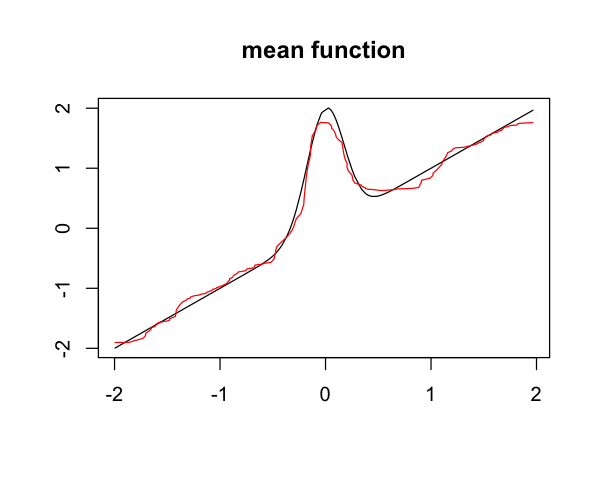plot(x, sigma.t^2, xlab = "", ylab = "", type = "l", main = "var function") lines(x, var.est[1:200], col = 2)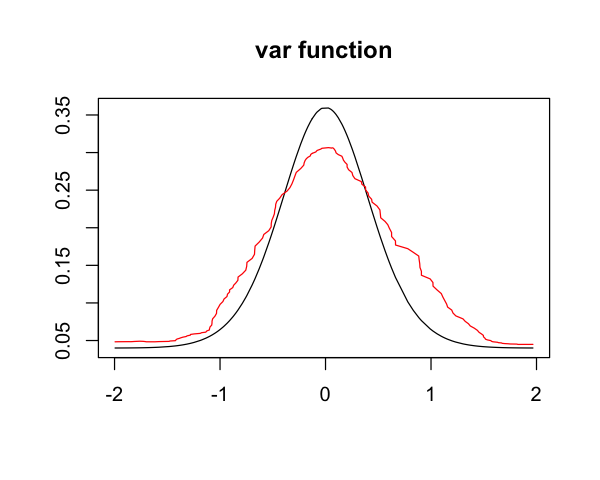## Treasury Bill example from Fan & Yao (1998) We also apply SMASH to the dataset in Example 1 of the Fan & Yao (1998) paper. To take into account replicates, we take the median of the response at the same point. The results are shown below. data(treas) y = ar(treas,FALSE,5)$res
y = y[!is.na(y)]
x = sort(treas[5:(length(treas) - 1)])
y = y[order(treas[5:(length(treas) - 1)])]

Plot the Treasury bill data.

plot(x, y)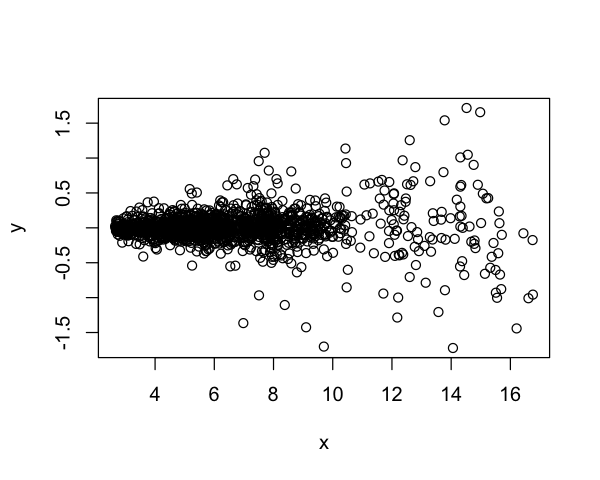## Average replicate data

x.mod = unique(x)
y.mod = 0
for (i in 1:length(x.mod)) {
y.mod[i] = median(y[x == x.mod[i]])
}
y.exp = c(y.mod, y.mod[length(y.mod):(2 * length(y.mod) - 2^10 + 1)])
y.final = c(y.exp, y.exp[length(y.exp):1])
y.est = smash.gaus(y.final)
y.est.var = smash.gaus(y.final, v.est = TRUE) 

More plots of the data.

par(mfrow = c(2, 2))
plot(treas, xlab = "year", ylab = "interest rate", type = "l")
plot(x, y)
lines(x.mod, y.est[1:(length(y.mod))], xlab = "X", ylab = "Y", col = 2)
plot(x.mod, y.est[1:(length(y.mod))], xlab = "X", ylab = "Y", type = "l",
ylim = c(-0.3, 0.3))
plot(x.mod, sqrt(y.est.var[1:(length(y.mod))]), xlab = "X",
ylab = "volatility", type = "l")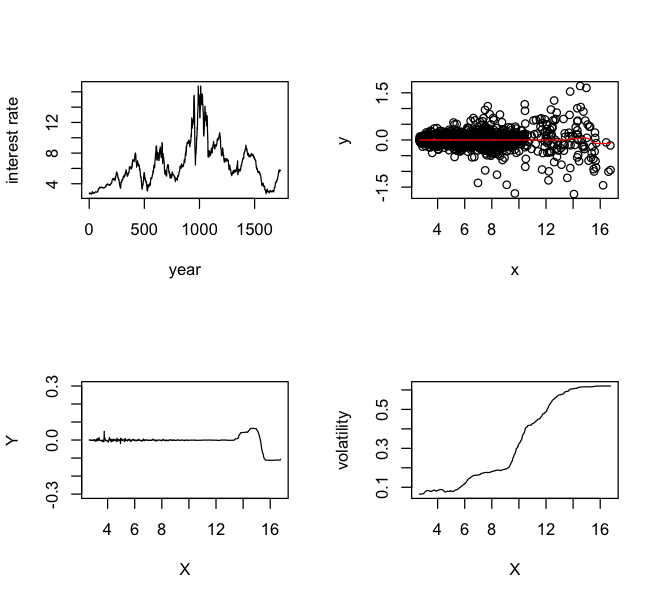## Heavisine example from Delouille et al (2004)

We now examine the example from Delouille et al. (2004). Specifically, we compare with the heavisine function used in section 6, as the actual function is readily available. The results for $$n=200$$ for both the homoskedastic and the heteroskedastic cases are given.

n = 200
mse.hsm.c = 0
mse.hsm.n = 0
for (i in 1:500) {
xt = sort(rnorm(n, 0.5, 0.2))
xt = (xt - min(xt))/(max(xt) - min(xt))

mu.hsm = 4 * sin(4 * pi * xt) - 2 * sign(xt - 0.3) - 2 * sign(0.72 - xt)

mu.t = mu.hsm
var1 = rep(1, n)
var2 = (xt <= 0.5) + 2 * (xt > 0.5)
sigma.ini = sqrt(var1)
rsnr = sqrt(4)
sigma.t = sigma.ini/mean(sigma.ini) * sd(mu.t)/rsnr^2

y = rnorm(n, mu.t, sigma.t)
y.exp = c(y, y[200:145])
y.final = c(y.exp, y.exp[256:1])

mu.est <- smash.gaus(y.final)
mu.est = mu.est[1:n]
mse.hsm.c[i] = mse(mu.est, mu.t)

sigma.ini = sqrt(var2)
rsnr = sqrt(4)
sigma.t = sigma.ini/mean(sigma.ini) * sd(mu.t)/rsnr^2

y = rnorm(n, mu.t, sigma.t)
y.exp = c(y, y[200:145])
y.final = c(y.exp, y.exp[256:1])

mu.est <- smash.gaus(y.final)
mu.est = mu.est[1:n]
mse.hsm.n[i] = mse(mu.est, mu.t)
}

sqrt(quantile(mse.hsm.c, seq(0.25, 0.75, 0.25)))
sqrt(quantile(mse.hsm.n, seq(0.25, 0.75, 0.25)))
#    25%    50%    75%
# 0.3705 0.4089 0.4411
#    25%    50%    75%
# 0.3741 0.4189 0.4589

## Motorcycle example from Delouille et al (2004)

We also look at the motorcycle in the same paper. We plot the data points and fit, as well as the estimated variances.

data(mcycle)
x.ini = sort(mcycle$times) y.ini = mcycle$accel[order(mcycle\$times)]

x = unique(x.ini)
y = 0
for (i in 1:length(x)) {
y[i] = median(y.ini[x.ini == x[i]])
}

y.exp = c(y, y[length(y):(2 * length(y) - 128 + 1)])
y.final = c(y.exp, y.exp[length(y.exp):1])

y.est = smash.gaus(y.final)
y.est = y.est[1:length(y)]
y.var.est = smash.gaus(y.final, v.est = TRUE)
y.var.est = y.var.est[1:length(y)] 

## Session info

This is the version of R and the packages that were used to generate the results shown above.

sessionInfo()
# R version 3.4.3 (2017-11-30)
# Platform: x86_64-apple-darwin15.6.0 (64-bit)
# Running under: macOS High Sierra 10.13.4
#
# Matrix products: default
# BLAS: /Library/Frameworks/R.framework/Versions/3.4/Resources/lib/libRblas.0.dylib
# LAPACK: /Library/Frameworks/R.framework/Versions/3.4/Resources/lib/libRlapack.dylib
#
# locale:
#  en_US.UTF-8/en_US.UTF-8/en_US.UTF-8/C/en_US.UTF-8/en_US.UTF-8
#
# attached base packages:
#  stats     graphics  grDevices utils     datasets  methods   base
#
# other attached packages:
#  wavethresh_4.6.8    EbayesThresh_1.4-12 smashr_1.1-7
#  MASS_7.3-48         rmarkdown_1.9
#
# loaded via a namespace (and not attached):
#   Rcpp_0.12.16        knitr_1.20          magrittr_1.5
#   REBayes_1.3-1       doParallel_1.0.11   pscl_1.5.2
#   SQUAREM_2017.10-1   lattice_0.20-35     foreach_1.4.4
#  highr_0.6           ashr_2.2-7          stringr_1.3.0
#  caTools_1.17.1      tools_3.4.3         parallel_3.4.3
#  grid_3.4.3          data.table_1.10.4-3 htmltools_0.3.6
#  iterators_1.0.9     assertthat_0.2.0    yaml_2.1.18
#  rprojroot_1.3-2     digest_0.6.15       Matrix_1.2-12
#  bitops_1.0-6        codetools_0.2-15    evaluate_0.10.1
#  stringi_1.1.7       compiler_3.4.3      Rmosek_8.0.69
#  backports_1.1.2     truncnorm_1.0-8